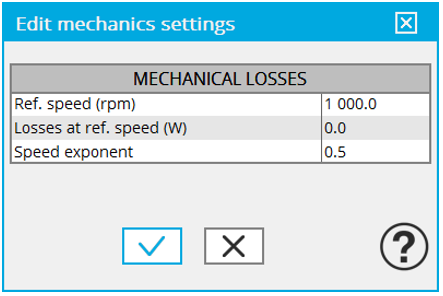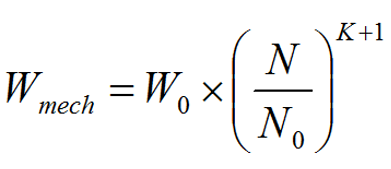# Mechanical loss model parameters

## Mechanical loss model settings

The definition of mechanical loss computation model is available only for some tests.User inputs for defining the mechanical losses

Mechanical loss model is considered as:The following input parameters are needed:

• “ speed ” (Reference speed) – N 0
• “ Losses at ref. speed ” (mechanical losses at reference speed) – W 0
• “ Exponent ” (Speed exponent) - K
• Current speed “N” for which the mechanical losses “W mech ” must be computed